Next: Momentum Conservation Up: Mathematical Models of Fluid Previous: Mass Conservation

# Convective Time Derivative

The quantity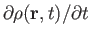, appearing in Equation (1.37), represents the time derivative of the fluid mass density at the fixed point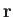. Suppose thatis the instantaneous fluid velocity at the same point. It follows that the time derivative of the density, as seen in a frame of reference which is instantaneously co-moving with the fluid at point, is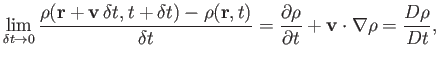(1.38)

where we have Taylor expanded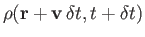up to first order in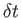, and where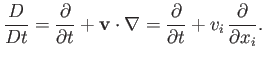(1.39)

Clearly, the so-called convective time derivative,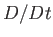, represents the time derivative seen in the local rest frame of the fluid.

The continuity equation (1.37) can be rewritten in the form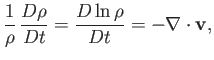(1.40)

because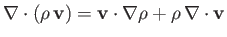. [See Equation (A.174).] Consider a volume elementthat is co-moving with the fluid. In general, as the element is convected by the fluid its volume changes. In fact, it is easily seen that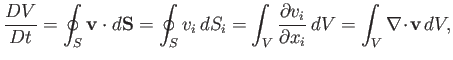(1.41)

whereis the bounding surface of the element, and use has been made of the divergence theorem. In the limit that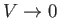, and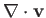is approximately constant across the element, we obtain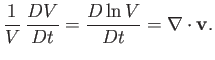(1.42)

Hence, we conclude that the divergence of the fluid velocity at a given point in space specifies the fractional rate of increase in the volume of an infinitesimal co-moving fluid element at that point.Next: Momentum Conservation Up: Mathematical Models of Fluid Previous: Mass Conservation
Richard Fitzpatrick 2016-03-31